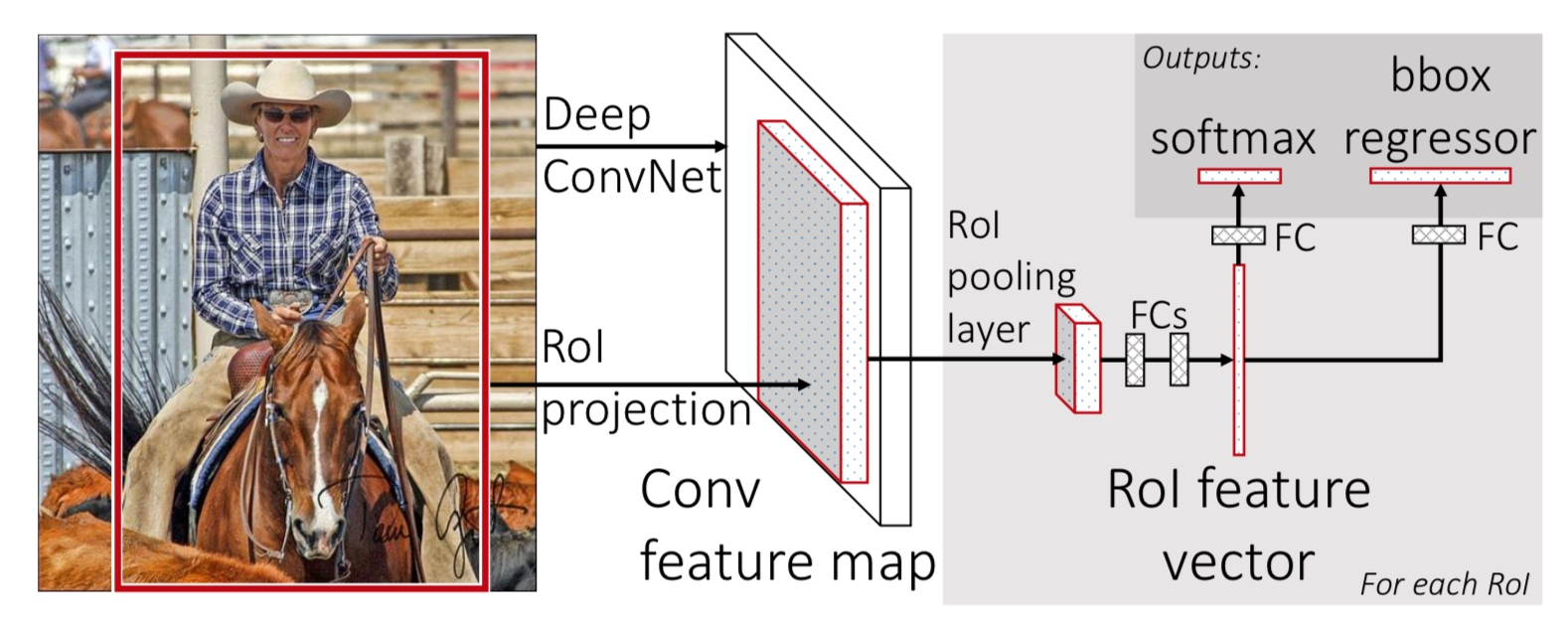# CNN的目标检测概述（三）

## 三、Fast R-CNN

### 1）R-CNN与SPPNet的不足

R-CNN在目标检测中有很好的准确率，但是这个方法本身仍有很多的问题。

1. 训练过程是多级的。 R-CNN的训练分成三个部分，首先是 finetune 一个网络（目标检测的类别和ImageNet不一样）。之后是使用SVM进行目标的分类的训练。最后是使用 feature map 来进行目标的 bounding-box 的回归训练。
2. 训练过程费时费空间。 SVM和回归两个任务，需要存储目标的特征，需要很多空间。网络的训练过程很慢。
3. 测试速度太慢。 需要对每个 proposal 进行前馈，耗时太长。速度只有47s / image。

SPPNet 可以加速 R-CNN，在 SPPNet 中，在卷积的部分直接前馈整张图片，之后对于每个 proposal，计算出它在 feature map 上的位置，然后使用 SPP Pooling 的方式，得到定长的特征向量。使得测试时间大大缩短。但 SPPNet 也有很多的问题。首先，SPPNet 在训练的过程和 R-CNN 几乎相同，因此有上面所有的问题，其次，SPPNet 只训练的网络最后的FC层，这样，前面的卷积的部分就没有参与训练。

### 2）Fast R-CNN的主要贡献

1. 更好的检测效果（mAP）
2. 训练是单步的，使用多任务loss
3. 所有的网络的参数都可以训练和更新
4. 不需要存储特征

### 3）Fast R-CNN的训练

Fast R-CNN 的结构如下：#### 1 RoI Pooling层

RoI Pooling 层是一种简化版本的 SPP Pooling 层。他的计算方式如下：

#### 2 使用预训练的网络

1. 将最后一个 max pooling 层替换成 RoI Pooling 层。输出固定的大小，并且这个大小和网络的全连接的输入要相匹配。（看样子，这里还想复用之前的FC层的参数）
2. 网络的最后的FC层，替换成两并列的FC。一个用于输出新的类别（K+1），另一个用来输出回归的结果（4K）。
3. 网络的输入变成两个，一个是图像数据（N个），另一个是 RoI（R个）。

#### 3 Fine-tuning for detection

Fast R-CNN 中，训练的样本是整张图像，网络的卷积部分可以处理任意尺度的输入，因此可以一次性得到整张图像的 feature map。之后，根据输入的 RoI 的位置（也就是之前的 Proposal），对每个区域在 feature map 上进行 RoI Pooling，得到固定大小的输出。最终经过若干个全连接，得到分类和回归的输出，监督训练。从而使得检测任务可以做到 one stage。整个过程包括：Lossmini-batch 采样策略，RoI Pooling 层的后馈以及 SGD 的超参数。下面专门依次介绍。

### 4）Fine-tuning for detection

$L(p,k^{*},t,t^{*})=L_{cls}(p,k^{*})+\lambda[k^{*}\ge1]L_{loc}(t,t^{*})$

$L_{loc}(t, t^{*}) = \sum_{i \in {x,y,w,h}} smooth_{L1}(t_{i}, t^{*}_{i})$

$smooth_{L1}(x) = \left\{\begin{matrix} 0.5x^{2} & if |x| < 1 \\ |x|-0.5 & otherwise \end{matrix}\right.$

$G=\{G_{x},G_{y},G_{w},G_{h}\}$

$\begin{matrix} t_{x} = \frac{G_{x} - P_{x}}{P_{w}} \\ t_{y} = \frac{G_{y} - P_{y}}{P_{h}} \\ t_{w} = \log{\frac{G_{w}}{P_{w}}} \\ t_{h} = \log{\frac{G_{h}}{P_{h}}} \end{matrix}$

#### 4 SGD 超参数

The fully-connected layers used for softmax classification and bounding-box regression are initialized randomly from a zero-mean Gaussian distribution with standard deviations 0.01 and 0.001, respectively. All layers use a per-layer learning rate of 1 for weights and 2 for biases (following standard practice) and a global learning rate of 0.001. When training on VOC07 or VOC12 trainval we run SGD for 30k mini-batch iterations, and then lower the learning rate to 0.0001 and train for another 10k iterations. When we train on larger datasets, we run SGD for more iterations, as described later. A momentum term with weight 0.9 and weight decay factor of 0.0005 are used in all experiments.

### 6）SVD分解，压缩检测

$W \approx U\Sigma _{t}V^{T}$

### 7）个人总结

Fast R-CNN 中，将目标检测的任务集成为一个清晰的框架，极大的简化了目标检测的训练过程，同时在速度和精度上均得到的很好的保证。其思想影响了之后的各种改进版本的目标检测算法。真的十分值得仔细的阅读。